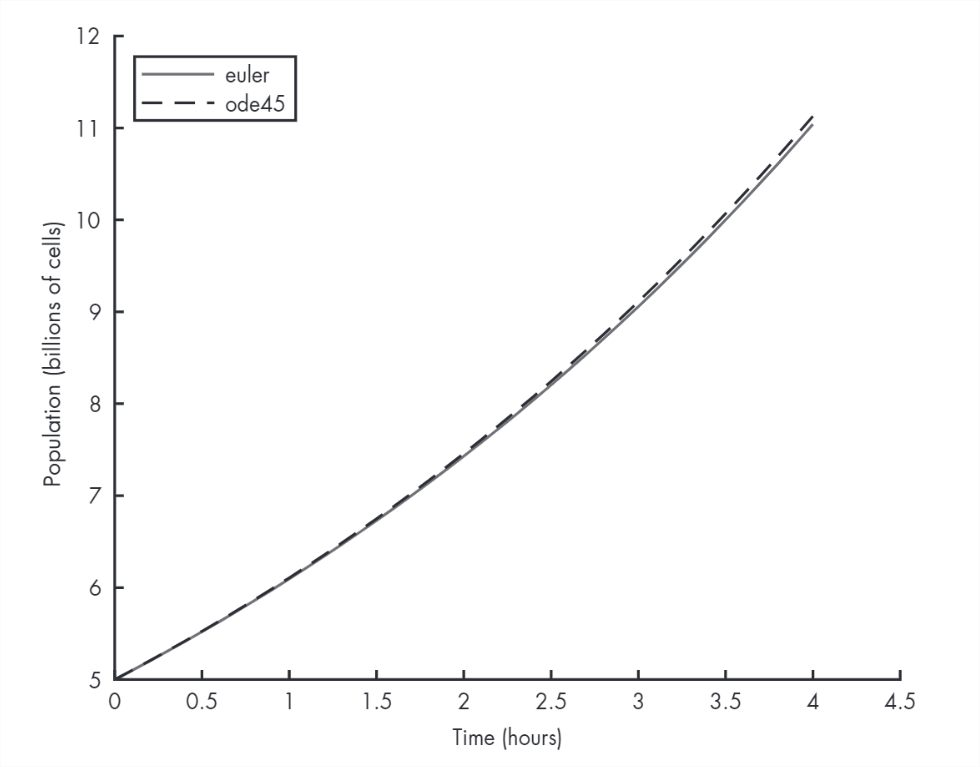# 19.5: Solving ODEs with ode45

$$\newcommand{\vecs}{\overset { \rightharpoonup} {\mathbf{#1}} }$$ $$\newcommand{\vecd}{\overset{-\!-\!\rightharpoonup}{\vphantom{a}\smash {#1}}}$$$$\newcommand{\id}{\mathrm{id}}$$ $$\newcommand{\Span}{\mathrm{span}}$$ $$\newcommand{\kernel}{\mathrm{null}\,}$$ $$\newcommand{\range}{\mathrm{range}\,}$$ $$\newcommand{\RealPart}{\mathrm{Re}}$$ $$\newcommand{\ImaginaryPart}{\mathrm{Im}}$$ $$\newcommand{\Argument}{\mathrm{Arg}}$$ $$\newcommand{\norm}{\| #1 \|}$$ $$\newcommand{\inner}{\langle #1, #2 \rangle}$$ $$\newcommand{\Span}{\mathrm{span}}$$ $$\newcommand{\id}{\mathrm{id}}$$ $$\newcommand{\Span}{\mathrm{span}}$$ $$\newcommand{\kernel}{\mathrm{null}\,}$$ $$\newcommand{\range}{\mathrm{range}\,}$$ $$\newcommand{\RealPart}{\mathrm{Re}}$$ $$\newcommand{\ImaginaryPart}{\mathrm{Im}}$$ $$\newcommand{\Argument}{\mathrm{Arg}}$$ $$\newcommand{\norm}{\| #1 \|}$$ $$\newcommand{\inner}{\langle #1, #2 \rangle}$$ $$\newcommand{\Span}{\mathrm{span}}$$$$\newcommand{\AA}{\unicode[.8,0]{x212B}}$$

A limitation of Euler’s method is that it assumes that the derivative is constant between time steps, and that’s not generally true. Fortunately, there are better methods that estimate the derivative between time steps, and they are much more accurate.

MATLAB provides a function called ode45 that implements one of these methods. In this section I’ll explain how to use it; you can read more about how it works in “” on page .

In order to use ode45, you have to write a function that evaluates $$dy/dt$$ as a function of $$t$$ and $$y$$. Fortunately, we already have one, called rate_func:

function res = rate_func(t, y)
a = 0.2;
dydt = a * y;
res = dydt;
end

We can call ode45 from the Command Window like this:

[T, Y] = ode45(@rate_func, [0, 4], 5);
plot(T, Y)

The first argument is a function handle, as we saw in Chapter . The second argument is the time interval where we want to evaluate the solution; in this case the interval is from $$t=0$$ to $$t=4$$ hours. The third argument is the initial population, 5 billion cells.

The ode45 function is the first function we’ve seen that returns two output variables. In order to store them, we have to assign them to two variables, T and Y. Figure 9.2 shows the results.Figure 9.2: Solutions to a simple differential equation using Euler’s method and ode45

The solid line is the estimate we computed with Euler’s method; the dashed line is the solution from ode45.

For the first 2–3 hours, the two solutions are visually indistinguishable. During the last hour, they diverge slightly; at 4 hours, the difference is less than 1 percent.

For many purposes, the difference between Euler’s method and ode45 is the least of our worries. In this example, we probably don’t know the initial population with perfect accuracy or the growth constant, a. Also, the assumption that the growth rate only depends on population is probably not true. Any of these modeling errors could be bigger than 1 percent.

However, for some problems, Euler’s method can be off by a lot more than 1 percent. In those cases ode45 is almost always more accurate, for two reasons: first, it computes the rate function several times per time step; second, if the time step is too big, ode45 can detect the problem and shrink the time step. For more details, see “How ode45 Works” on page .

This page titled 19.5: Solving ODEs with ode45 is shared under a CC BY-NC-SA 4.0 license and was authored, remixed, and/or curated by Allen B. Downey (Green Tea Press) via source content that was edited to the style and standards of the LibreTexts platform; a detailed edit history is available upon request.